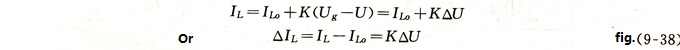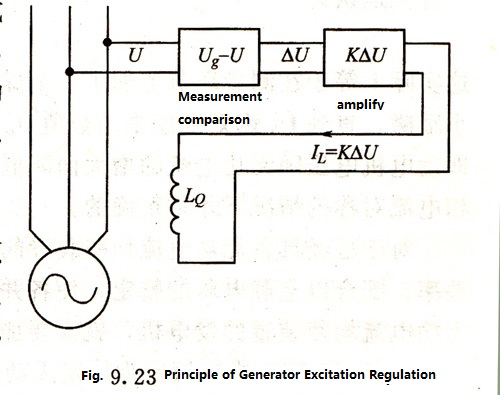# Automatic Distribution of Reactive Current for Diesel Generator

Jan. 17, 2019

## Automatic distribution of reactive current - regulation principle of regulator

It is not difficult to see that in order to ensure the uniform distribution of reactive current, two methods can be used in principle: one is to let the parallel running generator have the same voltage regulation characteristics and the necessary adjustment rate; Another method is to directly measure the reactive current deviation (Ik,) of each generator and then increase or decrease the generator excitation according to the size and symbol of Ik. A device using the former method is called an automatic reactive current distribution device. In order to ensure the uniform distribution of reactive power, these two methods are mostly used for regulating voltage deviations.

The so-called regulator deviation is simply adjusted, that is, the output of the voltage regulator - the excitation current is proportional to the voltage deviation of the generator, ieWhere Ug is the given value of the voltage regulation;

U——the actual voltage of the generator;

K——The proportional coefficient of the regulator, or closed loop magnification.

Figure 9. 23 is the schematic diagram of this voltage regulator. In order to facilitate analysis, the measurement and comparison link of the regulator is separately framed. The principle of this link is that the input generator terminal voltage is changed after a certain change (for example, transformer, rectifier), Compare with a given value, such as a voltage stabilizer, and then output a signal AU. that is proportional to the voltage deviation of the generator The excitation current output by the voltage regulator is proportional to the voltage deviation signal in a certain range. There are many kinds of regulating devices, such as thyristor voltage regulator, self-excitation constant voltage device with corrector, carbon resistive voltage regulator and so on. However, if only to discuss the problem of reactive load distribution, as long as we grasp the basic laws between the input (measurement link) and output (excitation current) of this type of voltage regulator, we can not delve into the wide variety of them. A complex intermediate link. Generally speaking, it is not difficult to do this.

### Two points should be highlighted for the characteristics of this type of voltage regulating system.

1. This type of voltage regulator is, in principle, a differential regulator, that is, for some reason (such as increased load current, higher temperature of the excitation winding, etc.) increases the voltage deviation U of the generator, thus changing the excitation current accordingly, In order to reduce the voltage deviation, the voltage deviation of the generator can not be completely eliminated, because the elimination of U also eliminates the basis of regulating the excitation current, so the voltage regulator must have differential characteristics. Nevertheless, in order to ensure the accuracy and rapidity of voltage regulation, such systems always have a very high magnification K, that is, a small change in U can make a strong enough change in the excitation current to maintain the generator voltage close to a given value. Therefore, for the reactive power distribution, this natural adjustment rate can be said to be insignificant, it can still be regarded as a no-difference characteristic. That is, the voltage regulator can be approximately considered to eliminate voltage deviation.2. The so-called voltage regulator eliminates voltage deviation, which refers to the voltage deviation signal that the measuring element input to it. This signal can be the actual voltage deviation of the generator or not the actual voltage deviation of the generator. For example, when the generator voltage is normal, a voltage is artificially superimposed on the input end of the measuring element to cause the deviation signal U, and the voltage regulator will change the excitation of the generator and finally make U ≈ 0. However, the generator voltage deviates from the given value, which is basically equal to the signal voltage superimposed on the measuring element. The principle of the reactive current adjusting device is to add a voltage signal proportional to the reactive current in the input of the measuring element, so that the voltage regulator can reduce the voltage of the generator and obtain the differential voltage regulation characteristic.